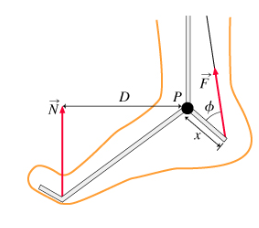# Problem: Find TF, the torque about point P due to the force applied by the Achilles' tendon. Express your answer in terms of F, phi, and x.

###### FREE Expert Solution

Let the magnitude of the applied force be F, and the distance between the line of action and axis of rotation be r.

Torque is then given as:

$\overline{){\mathbf{\tau }}{\mathbf{=}}{\mathbf{F}}{\mathbf{×}}{\mathbf{r}}}$

The line of rotation is along the direction of the force applied. The axis of rotation is at P.

r = x sin ɸ

87% (393 ratings)###### Problem Details

Find TF, the torque about point P due to the force applied by the Achilles' tendon.